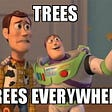# Logistic Regression

1. Introduction:

Logistic Regression is one of the most popular statistical models which is used for classification. It comes under a supervised machine learning model, which learns from the labeled data (i.e. given input and output for training).

Logistic Regression can be used to do either Binary (with 2 classes, for example, pass/fail, spam/not-spam) classification or Multi-Class (with 3 or more classes, example Food texture: Crunchy, Mushy, Crispy or Income level: low income, middle income, high income) classification.

In this article, I will focus on binary classification using Logistic Regression.

2. Use of Linear Regression for classification issue:

You might be thinking, why can’t we use Linear Regression for classification issues as well?

Let’s consider an example where x: age and y: owns a house or not. This is a classification problem in which we need to predict whether a person owns a house or not based on his age.

Can we use Linear Regression in such a classification problem?

Let’s use Linear Regression to separate the data into 2 groups with thresholds. All the data points below the threshold (0.5) will be classified as not owning the house, and all data points above the threshold (0.5) will be classified as owning the house.

Is the above solution working correctly or not, let’s test with some scenarios:

1. Suppose we got some new data-point on the extreme right side, suddenly we see that the slope of the line changes and which changes the threshold of the model. So this is the issue that our threshold of Age can not be changed in a predicting algorithm.

2. The second issue with applying Linear Regression to classification issues is that when we extend the linear regression line, it gives values more than 1 or less than 0, and we don’t know what does values are greater than 1 and smaller than 0 means.

3. Why Logistic Regression has term Regression in it:

Logistic regression is a generalized linear model and it uses the same basic formula of linear regression. Even though the name suggests regression, but Logistic Regression is a classification model, because the underlying assumption for Linear Regression and Logistic Regression is the same. We need a squashing function that can convert the continuous values into 1, 0. And which is not affected by outliers. For which, we use Logistic or Sigmoid function:

4. The cost function for Logistic Regression:

For Linear Regression, the cost function we use is the Mean Squared Error, which is the average of the summation of the square of the difference between predicted(ŷi) and the actual(yi) values:

This is a continous and convex function as shown below, and it can be optimized using the Gradient Descent algorithm:

In Logistic Regression the optimization function Q(z) is a nonlinear function(Q(z)=1​/1+ e-z), if we put this in the above Mean Squared Error equation, it will give a non-convex function.

If we try to use the cost function of the linear regression in Logistic Regression then it would be of no use as it would end up being a non-convex function with multiple local minimas, in which it would be very difficult to minimize the cost value and find the global minimum using Gradient Descent.

Hence, we use different cost functions for Logistic Regression, which is Log Loss or Binary Cross Entropy.

5. Log Loss with an example:

Below are the 3 steps to find Log Loss:

1. Find corrected probabilities
2. Take a log of corrected probabilities
3. Take the negative average of the values we get in the 2nd step

Log Loss is the negative average of the log of corrected predicted probabilities for each instance in the dataset.

By default, the output of the logistics regression model is the probability of the sample being positive (or class 1), for example:

Step1: Calculate Corrected Probabilities:

If the actual class is 1, the corrected probability will be the same as the predicted probability otherwise, it would be (1 - predicted) probability.

Step2: Calculate the log of corrected probabilities for each row:

Step3: Take the negative average of the values we get in the 2nd step using the below formula:

Log values are negative, so to handle a negative sign, we take the negative average of these values to maintain a common convention that lower loss scores are better.

6. Log Loss cost function for Logistic Regression:

Intuitively, we want to assign more punishment when predicting 1 while the actual is 0 and when predicting 0 while the actual is 1. The same thing happened in the log loss function. Below is the formula:

When the actual class (y) = 1, second term in the formula would be 0

When the actual class (y) = 0, first term in the formula would be 0

In the below diagram left side, when the actual class (y) = 1 and the predicted probability (ŷ) is close to 1, the loss is less and when the predicted probability (ŷ) is close to 0, the loss approaches infinity.

In the diagram right side, when the actual class (y) = 0, and the predicted probability (ŷ) is close to 0, the loss is less and when the predicted probability (ŷ) is close to 1, the loss approaches to infinity.

7. End Notes:

Please check my next blog for more details on the practical implementation of Logistic Regression from scratch without using sklearn, that would give you more understanding of how this model actually works.

8. References:

--

--

--

## More from Heena Sharma

Love podcasts or audiobooks? Learn on the go with our new app.

## Implement a simple neural network in C# .NET — Part 2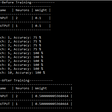## How to build your machine learning application## Illustrated Guide to Transformers- Step by Step Explanation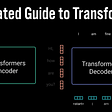## The Curse of Dimensionality## Why You Should Use Convolutions in Your Next Neural Net — Using TensorFlow## Understanding Dataset Shift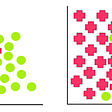## Object Detection State of the Art 2022## Understanding of Optuna-A Machine Learning Hyperparameter Optimization Framework## Linear Regression## Machine Learning 101: All things Regression## Simple Linear Regression Simplified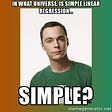## CART Method(Classification And Regression Trees) in Decision Tree